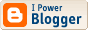## A Python Quicky - a function to handle user input (command line)

This is perhaps more a note to self, but of course others are welcome to use and improve on the function.

`#!/usr/bin/pythonimport tracebackimport redef UserInput( question="> ", input_type="numeric", regex="^\d{1}\$", action_on_fail="retry", help_on_error="\tYou need to supply exactly 1 numeric digit from 0 to 9. Press CTRL+C to quit." ): quit  = 0 userinput = "" while quit == 0:  try:   if input_type == "numeric":    userinput = str( input( str( question ) ) )    else:    userinput = str( raw_input( str( question ) ) )  except:   print "ERROR - Exception: "   print traceback.format_exc()   # Validate the input  if re.compile( regex ).search( userinput ):   quit = 1  else:   print "ERROR - Input validation failed."   print str( help_on_error )   if action_on_fail != "retry":    userinput = ""    quit = 1   else:    print "" return userinputprint "Testing user input...\n\n"born = UserInput( "In which year were you born? ", "numeric", "^\d{4}\$", "retry", "Enter a year valid 4 digit year" )name = UserInput( "What is your name? ", "alpha", "^\w{3,32}\$", "retry", "A valid name contains only alpha characters and is between 3 and 32 characters long" )if int( born ) < 1980: print "Hi " + str( name ) + " - You are old :-)"else: print str( name ) + " - you're a youngster..."`

As you can see, I have used normal regex validation, so nothing too fancy.

Comments: Post a Comment

<< Home